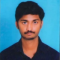## Solve the following equation and also verify your solution:$\frac{2}{3}(x-5)-\frac{1}{4}(x-2)=\frac{9}{2}$Updated on 13-Apr-2023 23:02:58
Given:The given equation is $\frac{2}{3}(x-5)-\frac{1}{4}(x-2)=\frac{9}{2}$.To do:We have to solve the given equation and verify the solution.Solution:To verify the solution we have to find the value of the variable and substitute it in the equation. Find the value of LHS and the value of RHS and check whether both are equal.The given equation is $\frac{2}{3}(x-5)-\frac{1}{4}(x-2)=\frac{9}{2}$.$\frac{2(x-5)}{3}-\frac{1(x-2)}{4}=\frac{9}{2}$$\frac{2x-10}{3}-\frac{x-2}{4}=\frac{9}{2}LCM of denominators 3 and 4 is 12.\frac{(2x-10)\times4-(x-2)\times3}{12}=\frac{9}{2}$$\frac{8x-40-3x+6}{12}=\frac{9}{2}$$\frac{5x-34}{12}=\frac{9}{2}On cross multiplication, we get, (5x-34=\frac{9\times12}{2}$$5x-34=9\times6$$5x-34=54$$5x=54+34$$5x=88$$x=\frac{88}{5}$Verification:LHS $=\frac{2}{3}(x-5)-\frac{1}{4}(x-2)$$=\frac{2}{3}(\frac{88}{5}-5)-\frac{1}{4}(\frac{88}{5}-2)$$=\frac{2}{3}(\frac{88-5\times5}{5})-\frac{1}{4}(\frac{88-2\times5}{5})$$=\frac{2}{3}(\frac{88-25}{5})-\frac{1}{4}(\frac{88-10}{5})$$=\frac{2}{3}(\frac{63}{5})-\frac{1}{4}(\frac{78}{5})$$=\frac{2}{1}(\frac{21}{5})-\frac{1}{2}(\frac{39}{5})$$=\frac{42}{5}-\frac{39}{10}$$=\frac{42\times2-39}{10}$$=\frac{84-39}{10}$$=\frac{45}{10}$$=\frac{9}{2}$RHS $=\frac{9}{2}$LHS $=$ RHSHence verified.Read More

## Solve each of the following equations and also verify your solution:(i) $\frac{(2x-1)}{3}-\frac{(6x-2)}{5}=\frac{1}{3}$(ii) $13(y-4)-3(y-9)-5(y+4)=0$Updated on 13-Apr-2023 23:02:13

## Solve each of the following equations and also verify your solution:(i) $\frac{2x}{3}-\frac{3x}{8}=\frac{7}{12}$(ii) $(x+2)(x+3)+(x-3)(x-2)-2x(x+1)=0$Updated on 13-Apr-2023 23:00:44

## Divide:(i) $5x^3-15x^2+25x$ by $5x$(ii) $4z^3+6z^2-z$ by $\frac{-1}{2}z$(iii) $9x^2y-6xy+12xy^2$ by $\frac{-3}{2}xy$Updated on 13-Apr-2023 22:56:43